Conservation of Momentum

Momentum is conserved in any collision if the effect of any external forces present is negliable relative to the effect of the collision. Consider a collision as shown in Figure (6.1).Apply the impulse-momentum theorem to m1 and m2 separately,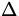t =p1 = m1v1f - m1v1i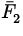t =p2 = m2v2f - m2v2i
where= the average force of m2 on m1 , and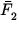= the average force of m1 on m2 . By Newton's third law F1(t) = - F2(t) which gives= -and so,
 (+)t = m1v1f - m1v1i + m2v2f - m2v2i = 0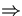p1f + p2f = p1i + p2i. (4)
This is the statement of the conservation of momentum.

Note:

• The system must be isolated: the affect of all external forces acting on m1 and m2 must be negligable.
• The conservation of momentum holds for a collision involving any number of objects: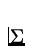=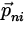. (5)
• Momentum is a vector, and each component is conserved separately. The equation for conservation of momentum really contains three equations, one for each dimension.Next: Collisions and Kinetic Energy Up: Momentum and Collisions Previous: Momentum and Impulse